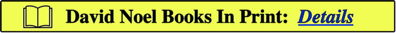Mass Gravity and Spin Gravity

BS806: Mass Gravity and Spin Gravity:

David Noel
<davidn@aoi.com.au>
Ben Franklin Centre for Theoretical Research
PO Box 27, Subiaco, WA 6008, Australia.

What is commonly called "Gravity" is now reasonably well understood. The basic law was derived by Isaac Newton back in 1687, and the usual formula quoted is as follows.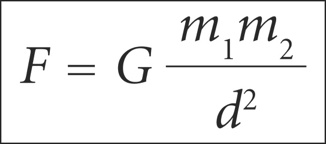. . . (1)
Figure BS806-F1. The standard Mass Gravity equation.

This equation states that the (attracting) gravitational force "F" between two bodies of mass "m1" and "m2 " is equal to the product of their masses, divided by the square of the distance "d" between them, multiplied by a constant "G". This is called the Gravitational Constant, and its value depends on the units used for the other terms.

In simple terms, gravity is higher between objects of higher mass, and lowers rapidly, the further they are apart.

In SI units, G has the value 6.67 x 10-11 Newtons kg-2 m2. The direction of the force is in a straight line between the two bodies and is attractive .

The Mass Gravity equation has proved its worth over several centuries, and there is no suggestion here that it may be inaccurate. But there is another sort of gravity, which we will call Spin Gravity, which is much less familiar. Spin Gravity applies between two objects which are rotating (they have spin), in addition to the normal Mass Gravity.

When two objects are rotating, they experience the normal gravitational force between them, in accordance with their masses and separation. But, in addition (provided that their axes and directions of rotation are not already exactly aligned), they experience further forces between them. These forces, here called Spin Gravity, work to make the two objects closer in their spin parameters.

This not a familiar area for most, although "tidal effects" have some relevance. In everyday science, the application most known about is when the "Slingshot Effect" or "Gravity Assist" is applied to space probes.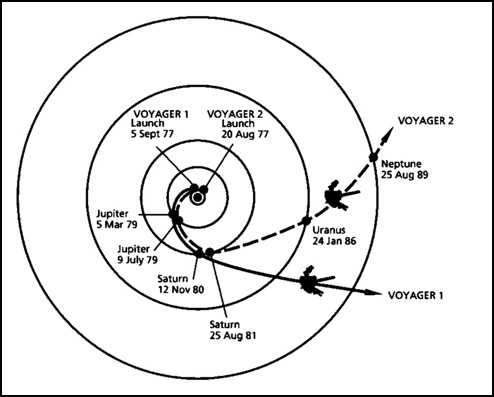Figure BS806-F2. Gravity assist and Voyager 1 and 2 spacecraft. From .

Both the Voyager 1 and Voyager 2 spacecraft were sent on specific paths past the planets Jupiter and Saturn to enable them to gain extra impetus from the slingshot effect, and take on new paths out of the Solar System. In these manoeuvres, the spacecraft gained energy from the rotational energy of the planets, useful for the spacecraft, but insignificant losses for the planets.

The following simple equation just says that when two objects exchange momentum (energy of movement), the total energy involved remains the same.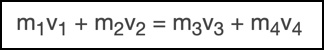Figure BS806-F3. Conservation of momentum equation. From .

Spin Gravity and Frame Dragging
An even less familiar aspect of Spin Gravity is an effect which stems from Albert Einstein's General Theory of Relativity, called Frame Dragging. Essentially this says that when an object is spinning in space, its motion encounters a tiny amount of drag from the influence of the part of the Universe which surrounds it.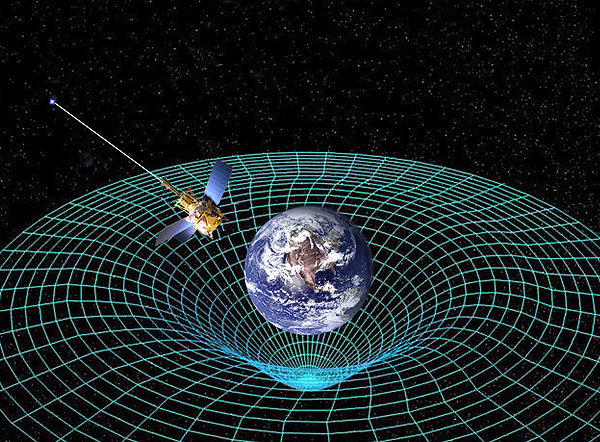Figure BS806-F4. Gravity Probe B satellite tested frame dragging. From .

The existence of Frame Dragging has been verified from measurements by a special satellite, called Gravity Probe B . Frame dragging has also been used to explain the detailed interaction between two massive stellar objects, such as black holes.

Generally speaking, Spin Gravity effects are difficult to detect in the real Universe, because the forces involved are very tiny compared to those of Mass Gravity. They only tend to show up when very large masses, spin rates, or time lapses apply.

Equatorial Forcing permeates the Universe
In 2014 I carried out an analysis of how the Solar System was formed, in "P1: The Cosmic Smog model for solar system formation, and the nature of 'Dark Matter'" , working from the assumption that our planets, moons, and asteroids were mostly formed independently of the Sun, and subsequently reorganized into their current conformation.

This analysis was extraordinarily fruitful, giving clear answers to many previously unanswered questions about the Universe, one dating back to Isaac Newton himself.

A currently-popular idea about how the Solar System formed is that the early Sun somehow became surrounded by a "Protoplanetary Disc", and that the planets condensed from this disc. This model is a dud. No physics-based reasoning has ever been produced as to why such a disc should be produced during star formation, or why it should rotate in the star's equatorial plane. Later we will see why this model gained plausibility.

In the new model , the Solar System's current planets and smaller bodies were formed independently from the Sun, and were initially fairly randomly placed. A gravitational effect called Equatorial Forcing, described in "XT806: What is Equatorial Forcing?"  very gradually normalized the orbits of the planets so that they orbited fairly closely to the plane of the Sun's equator.

This pattern was clearly evident from graphs of how bodies orbiting the Sun had their orbits closer and closer to the Sun's equatorial plane, the nearer they were to the Sun. Comets with orbits taking them far outside the Heliosphere (the edge of the Solar System), travel at any random angle to the plane. Most objects orbiting just inside the Heliosphere, such as the dwarf planets Haumea and Makemake, orbit within about 29 degrees of the plane. The true planets, all lying within the inner third of the Heliosphere, stray no further than 7 degrees from the plane.

The empirically identified force involved, here called Equatorial Forcing, is clearly a particular case of Spin Gravity. In Equatorial Forcing, a massive rotating central body exerts influence on smaller objects in its vicinity, causing their orbits around the central body to very slowly get closer to its own plane and direction of rotation.

With our Solar System, there is evidence of movement of orbiting bodies down into the Sun's equatorial plane in the Late Heavy Bombardment event . This event, which occurred about 4.1 to 3.8 billion years ago, was a time when the Moon and the planets were subjected to particularly heavy collisions with asteroids -- just as would be expected as orbits were normalized and more bodies were moving in the same plane.

The Equatorial Forcing explanation also answers Isaac Newton's question, as to why the Planets orbit in a plane, while comets travel at any inclination to this plane. The same mechanism also explains other features noted inside and outside the Solar System. A particularly striking example of this concerns the Rings of Saturn.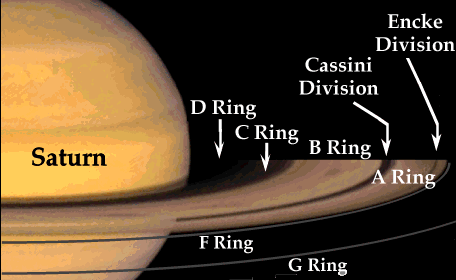Figure BS806-F5. The Rings of Saturn. From .

Surrounding Saturn there are very wide bands of circling minor objects, believed mostly balls of ice, snow and dust, perhaps only 10 metres across, which orbit directly above Saturn's equator. Do a Google search on why these rings have formed where they are, and you won't find a convincing answer. Equatorial Forcing provides this answer.

It should be understood that Equatorial Forcing is more obvious for smaller bodies, closer to the central one, and over long periods of time. It took around a billion years for the Sun's planets to come down into its equatorial plane. The Saturn ring objects are small and close, and conformed quickly. Moons around a planet orbit fairly close to the planet's own plane of rotation, which is clearly explained by Equatorial Forcing -- and note that there have never been suggestions of moons being formed in a "Proto-lunar" disc.

When it comes to the shapes of galaxies, older ones are typically discs. This is logically explained by the Equatorial Forcing exerted by the AGN (supermassive black hole) at the galaxy's centre.

In formation of a new solar system, spin gravity will inevitably work to bring the captured planetary objects down into the star's equatorial plane. They will then somewhat resemble a protoplanetary disc, hence they could be believed to have formed from a disc.

Formula for Spin Gravity
Following is an empirically derived equation for the gravitational forces due to spin gravity, a consequence of the rotation of the bodies involved.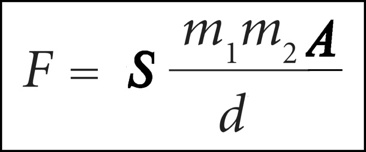. . . (2)
Figure BS806-F6. The Spin Gravity equation.

This equation has some similarity to the equation for Mass Gravity, and some significant differences. This equation states that the Spin-Gravitational force "F" between two rotating bodies of angular momentum "m1" and "m2 " is equal to the product of their angular momentums, multiplied by an Angular Fraction A, and divided by the distance "d" between them, all multiplied by a constant "S". This constant is called the Spin Gravitational Constant.

The basic differences between the Mass Gravity and Spin Gravity equations are that the Spin version uses the bodies' rotational energy (loosely equated with angular momentums) rather than their masses; it contains an additional term "A"; and the force F is inversely proportional to the distance separating the masses, rather than to the square of this distance.

The reasoning here is that Spin Gravity acts in two dimensions rather than three, and so will fall off directly with distance -- the force involved is spread over a plane, rather a three-dimensional space.

The Angular Fraction "A" represents the angle between the north-pole axes of the two rotating bodies, as a fraction of a half-circle. For example, if body A is rotating with its north pole directly up, and body B has its north pole pointing to the right, the Angular Fraction is 90/180 degrees, or 0.5 (see Figure F7 (a) ).

The Spin Gravity force is at its greatest when the north poles of the two bodies point in directly opposite direction, so "A" is 180/180, or 1 (unity). When the two bodies have their north poles pointing in exactly the same direction, "A" is zero, and there is no spin gravity force acting at all.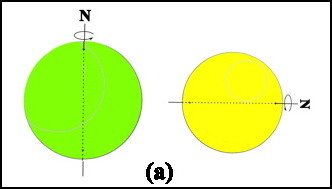.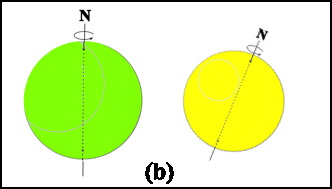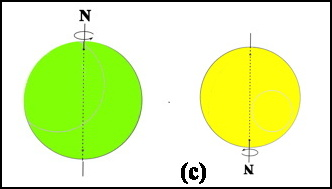.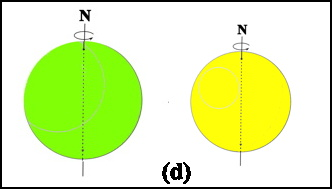Figure BS806-F7. (a) F is mid, A=0.5; (b) F is small, A=0.2; (c) F is maximum, A=1 ; (d) . A=0, so F=0.

Orbital resonance in planets and moons
When there are whole-number ratios between the times of planetary and moon orbits, this is called orbital resonance. Mass gravity explains the situation as it is now, but does not explain how it got there -- for this, spin gravity is needed.

One of the best-known resonances is between Neptune and Pluto -- for every 2 orbits Neptune makes round the Sun, Pluto makes 3. (This is one reason why, even though Pluto's orbit is eccentric and sometimes takes it closer to the Sun than Neptune, the two bodies do not collide -- resonance keeps Pluto distant from Neptune when in the same part of space).

Another interesting case is that of Pluto and its moons. Pluto and its large "moon" Charon actually form a "double dwarf planet" -- they have their centre of gravity outside Pluto, in the space between them. Around this joint centre of gravity orbit four more moons, Styx,Nix, Kerberos, and Hydra. The whole 6-body system is flat, and Pluto and Charon face each other, "tidally locked".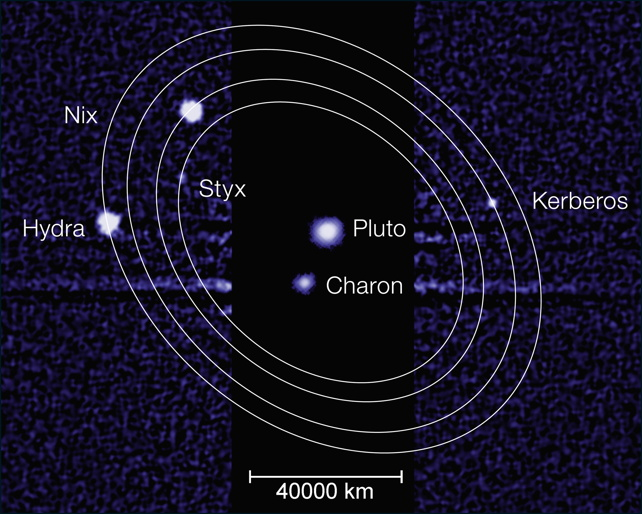Figure BS806-F8. Pluto and its moons. From .

Styx, Nix, and Hydra are thought to be in a 3-body orbital resonance with orbital periods in a ratio of 18:22:33; the respective ratio of orbits is 11:9:6 . The ratios should be exact when orbital precession is taken into account. Hydra and Nix are in a simple 2:3 resonance. Styx and Nix are in an 11:9 resonance, while the resonance between Styx and Hydra has a ratio of 11:6. This means that in a recurring cycle, there are 11 orbits of Styx for every 9 of Nix and 6 of Hydra.

All of the outer circumbinary moons are also close to mean motion resonance with the Charon-Pluto orbital period. Styx, Nix, Kerberos, and Hydra are in a 1:3:4:5:6 sequence of near resonances, with Styx approximately 5.4% from its resonance, Nix approximately 2.7%, Kerberos approximately 0.6%, and Hydra approximately 0.3% . There is a similar pattern, known as a three-body resonance, in the so-called Laplace resonance that links Jupiter's moons Io, Europa and Ganymede .

Mass and Spin Gravity in the Universe
Mass gravity tells us why planets and moons are where they are, but doesn't tell us how they got there, it has no explanation for resonance. Spin gravity supplies this explanation.

We can generalize the situation with a Proposition.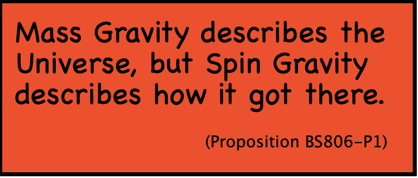Proposition BS806-P1.

Another comment might be that Mass Gravity builds the Universe, but Spin Gravity decorates it.

Black Holes and Vortex Stars
Recent work  has improved understanding of the class of objects called AGNs, or supermassive black holes, which lie at the heart of every galaxy. AGNs are the largest known of the series of Vortex Stars , which range upwards from White Dwarfs, Neutron Stars, and Stellar Black Holes.

The position of AGNs (short for Active Galactic Nucleus) at the top of the size scale means that they are objects which have such extreme properties that they may stray beyond the established behaviours of more everyday objects. AGNs may have millions of times the mass of our Sun, even billions of such masses.

AGNs also rotate extremely rapidly. The most rapid rates of rotation known to date occur in a type of AGN called a millisecond pulsar -- these have a rotational period smaller than about 10 milliseconds , and so rotate more than 100 times in a single second. Millisecond pulsars have been detected in radio, X-ray, and gamma ray parts of the electromagnetic spectrum.

Pulsar PSR J1748-2446ad, discovered in 2004, is, as of 2021, the fastest-spinning pulsar currently known, spinning 716 times per second.Figure BS806-F9. Artist's impression of a pulsar vortex star, with axial beams, fed by material drawn from a companion star. From .

Figure F9 shows an artistic impression of PSR J1023+0038, a binary system composed of a neutron star which tears matter off a companion star and forms an "accretion disk", and emits beams of visible light. The inset shows the pulses of visible light observed by the photometer SiFAP at the INAF - Telescopio Nazionale Galileo in Italy  -- one pulse per rotation.

All vortex stars are extremely dense objects, rotating extremely rapidly, which emit beams of radiation (and sometimes matter, such as protons) along their two axes.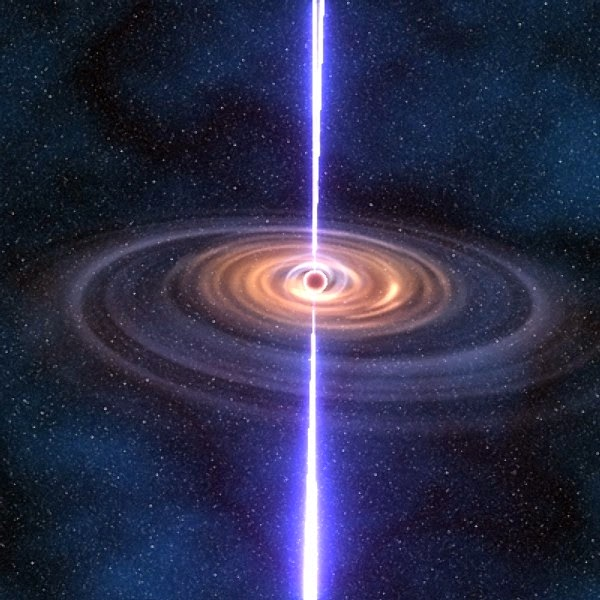Figure BS806-F10. Axial beams from neutron stars and black holes. From .

Figure F10 shows a conventional representation of a vortex star, such as a neutron star or a black hole, emitting beams along its axes. The star is shown surrounded by a rotating disc, called an accretion disc. There is no direct evidence that such a disc exists in real vortex stars -- but it gives a good visual image.

The axial beams depicted are real phenomena, but in actuality they are extremely narrow and tightly focussed, similar to laser beams, with very little spread. Every galaxy has an AGN vortex star at its centre, emitting these tight beams, though they are only very obvious when the galaxy plane is at 90 degrees to the observer. However the beams may strike intervening matter, and other sources of light will occur within the galaxy, which may then show structure.

The beams are oppositely polarized, according to the direction of rotation of the star, and may include radiation from all wavelengths of the electromagnetic spectrum, from low-energy radio waves, through visible light, up to high-energy x-rays. The more massive vortex stars may also produce matter streams, such as protons and electrons. These particles are charged, and so may lose beam tightness through interaction with intervening magnetic fields.

Some pulsars have an extremely short spin period of a few milliseconds and rotate hundreds of times every around their axis. According to . an object located at their equator would travel at an astonishing velocity of ten percent of the speed of light (if the vortex star's composition was uniform -- probably unlikely).

The nature of Mass
Although a depiction like that in Figure F10 gives a mind picture to use for black holes and other vortex stars, the actual nature of a black hole presents puzzles in comprehension. Vortex star objects of increasing magnitude imply greater masses, and even greater densities. The leaps in density on going up the scale are each of many orders of magnitude.

As an example, our Earth has a diameter of about 12,750 kilometres. If it had the density of neutron star material, its diameter would be about 330 metres. If made of black-hole density material, it would be only about 3 cm in diameter.

Densities of this order are hard to find explanation models to describe them. Neutron stars have the density of atomic nuclei, of neutrons, and so can be thought of as atoms stripped of their surrounding electron clouds and so existing as the nuclei alone. But what about black holes, how can we envisage the form of their immense masses, how can they be much denser than nuclei?

There is a tentative model which can be put forward for discussion. It relies on the famous Einstein equation, E=mc2, which says that the amount of energy, E, which can be derived from a given mass, m, is equal to the mass multiplied by the square of the speed of light.

An alternative reading of this equation says that Mass and Energy are equivalent, they are only alternative descriptions which can be applied to something. So the enormous rotational energies of black holes can be treated as their masses. While we have have no knowledge of any substance denser than an atomic nucleus, there is no theoretical limit to the rate of rotation of an object -- except perhaps when a rotating part approaches the speed of light.

So in this new model, the immense rotational energies existing in AGNs and other black holes expresses itself as their masses. In the Spin Gravity equation given above, the quantities m1 and m2 then represent spin masses, rather than angular momentums -- though these "spin" masses may, or may not, be different to what is usually taken as their mass.

We can again represent the situation with a Proposition.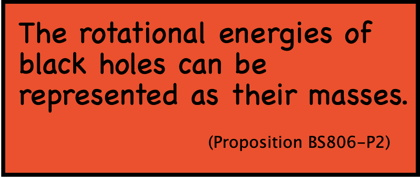Proposition BS806-P2.

Galaxy Rotation Curves and Dark Matter
During the 1930s, the brilliant Swiss-American astronomer Fritz Zwicky made observations of the rates at which parts of a galaxy distant from its centre were rotating. Normal Mass Gravity laws would lead to the expectation that the further the orbit of an object was from a gravitational centre, the slower it would travel. Orbital rates of Solar System planets, dwarf planets, and inner-system comets conform exactly with the formulas.

However, Zwicky found that after a certain distance was reached (for a galaxy like the Milky Way, about 40,000 light years out), measured orbital rates did not decrease with distance, but tended to level out.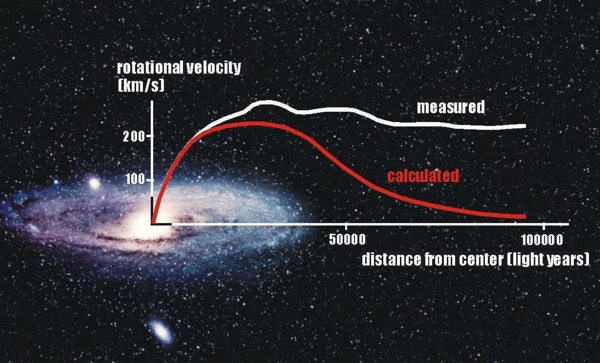Figure BS806-F11. How galaxy rotation speeds vary with distance from centre. From .

Zwicky suggested that this was because the spaces beyond the galaxy's visible boundaries contained a lot more matter, as bodies smaller than stars, which was not visible and was only detectable from its gravitational influence -- he called this "Dark Matter". Ever since his time, researchers have been searching for the nature and existence of this "Dark Matter", which has only recently been identified ( and ).

Here is some of what  has to say about the matter: "Astronomers have been searching for a mysterious substance called "dark matter" since the 1930s. Most scientists still believe it exists, despite many fruitless decades.

One reason why physicists have been trying to find dark matter is because of anomalies in the "galaxy rotation curve" data. Matter, such as gas and stars, tends to rotate around the centre of individual galaxies. The variation of this rotation speed if plotted against distance from the centre of the galaxy gives what is known as that galaxy's rotation curve.

Matter that orbits quite far away from the centre of the galaxy is expected to have a lower rotational speed than matter orbiting closer to the centre. But observations consistently show a "flattening" of the rotation curves -- in which speeds remain essentially constant beyond a certain distance from the galactic centre. Rather than challenge the theories of Newton and Einstein, it has been accepted that each galaxy must be surrounded by a vast halo consisting of dark matter (termed so because it cannot be directly observed)"
.

But now, the current treatment of Spin Gravity offers an alternative explanation for the shape of the Galaxy Rotation Curves.

It was noted earlier that with standard Mass Gravity, the gravitational effect falls off with the square of the distance involved, but with Spin Gravity, the effect reduces directly with distance.

In a galaxy, Mass Gravity is large and dominant, but at great distances, its effect falls off relatively sharply. Spin Gravity, on the other hand, is much weaker, but falls off much less rapidly. The suggestion is, that the point where the rotation curve flattens is the distance where Spin Gravity forces start to become more important than Mass Gravity forces.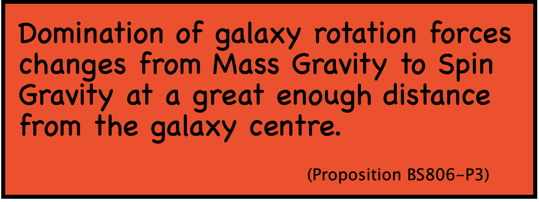Proposition BS806-P3.

If this Proposition proves to be valid, it gives a direct route to calculating the value of the Spin Gravity Constant "F" (in equations (1) and (2), where "d" has the same value in both equations).

* * * * * * * * * * * * * * * * * *. Gravitational Constant. https://astronomy.swin.edu.au/cosmos/g/Gravitational+Constant .
. The Slingshot Effect. https://nsb.wikidot.com/pl-9-2-3-4 .
. Stanford's Gravity Probe B confirms two Einstein theories. https://news.stanford.edu/news/2011/may/gravity-probe-mission-050411.html .
. David Noel. P1: The Cosmic Smog model for solar system formation, and the nature of 'Dark Matter'. http://aoi.com.au/bcw1/Cosmic/index.htm .
. David Noel. XT806: What is Equatorial Forcing?. http://www.aoi.com.au/Extracts/XT806.htm .
. Late Heavy Bombardment. https://en.wikipedia.org/wiki/Late_Heavy_Bombardment .
. Pluto's Moons Move in Synchrony. https://www.scientificamerican.com/article/pluto-s-moons-move-in-synchrony/ .
. Moons of Pluto. https://en.wikipedia.org/wiki/Moons_of_Pluto .
. David Noel. The Oort Cloud: Almost All the Universe. AOI Press, 2020. ISBN 9798614884314 .
. Millisecond pulsar. https://en.wikipedia.org/wiki/Millisecond_pulsar .
. Pulses of visible light from a millisecond pulsar. http://www.tng.iac.es/news/2017/10/16/pulsar/.
. Tibi Puiu. Peculiar pulsar slows down before "glitching". https://www.zmescience.com/science/peculiar-pulsar-slows-down-before-glitching/ .
. New theory of general relativity casts doubt on dark matter. https://theconversation.com/new-theory-of-general-relativity-casts-doubt-on-dark-matter-16446 .